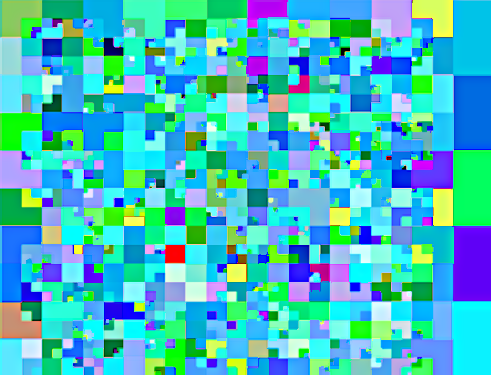Below are four C++ implementations of the region quadtree (the kind used for image compression, for example). The different implementations were made in an attempt to optimise construction of quadtrees. (For a tutorial on implementing region quadtrees, see Issue 26 [6.39 MB zip] of Dev.Mag).

• NaiveQuadtree is the straightforward implementation.
• AreaSumTableQuadtree uses a summed area table to perform fast calculations of the mean and variance of regions in the data grid.
• AugmentedAreaSumTableQuadtree is the same, except that the area sum table has an extra row and column of zeros to prevents if-then logic that slows it down and makes it tricky to understand.
• SimpleQuadtree is the same as AugmentedAreaSumTableQuadtree , except that no distinction is made (at a class level) between different node types.

The interfaces of all quadtrees are the same, but I did not want to extend from a base class. (Instead, a compile time check is performed on the classes, using boost concepts).

The results of the performance (on my machine!) of the trees are as follows:

 (milliseconds) N S AST AAST 3 4 4 3 64×64 14 14 14 13 128×128 55 52 55 52 256×256 229 233 218 214 512×512 950 1036 937 922 1024×1024 4064 4459 5396 3891

As it turns out, the different implementations do not differ significantly. Constructing the nodes takes long, and not so much the calculations necessary to determine whether a node should split or not, and what data should be in the node. Had I profiled properly before I started I would not have gone through this exercise…

Between these four implementations, the NaiveQuadtree is the one I recommend; I left in the other implementations for anyone interested.

The one good thing that came from this experiment is that I found out that using a zero-augmented summed area table can increase performance quite a bit. This is useful for max-filters and other algorithms that use these tables.